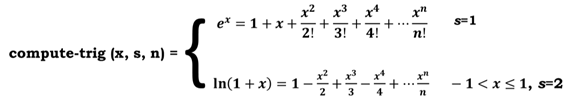COMP 348: Principles of Programming Languages Assignment 2 Solved

25.00 \$ 12.50 \$

Category:
Click Category Button to View Your Next Assignment | Homework

Description

Note: For this assignment, you can make use of all the in-built predicate functions like oddp, evenp, atom, etc. However, you can’t use any other utility functions such as length of list. You will need to use the “error” function, description is available at:

Question 1 (15 pts)

Write a lisp function mytable that takes in one argument x and prints the table for it. Your program should print an appropriate message for decimal as well as string values of x. Sample program output is shown below.

(mytable 2.3) Decimal values not allowed, please enter an integer

(mytable ‘dd) String values not allowed, please enter an integer

(mytable 5)

5 1      5

5 2      10

5 3      15

5 4      20

5 5      25

5 6      30

5 7      35

5 8      40

5 9 45 5 10 50

Question 2 (15 pts)

Given an input list of mixed items (such as: integers, decimals, characters, strings, or other nested lists). Write a lisp function that can compute sum of all the elements of the input list which are odd integers. Your program must be able to handle decimal values, character or string values, as the elements inside of its nested lists. Sample program output is shown below.

1. ‘((1 4) ((f 9.0 6) (5))) è 6
2. ‘((1 a b) ((f 9 6) (7))) è 17
3. ‘((1 ( ) 7)(((3 (g) 2)))) è 11
4. ‘((2 4 f a) (8) (w 6)) è 0
5. ‘(2 4 ( ) ((( ))) 5 ( ) 2) è 5

Question 3 (15 pts)

1. Compare these two functions (sum-list1 and sum-list2), what these function do?
2. Which one is more efficient or more readable? and why?

(defun sum-list1 (L)

(if (null L) 0

(+ (first L)

(sum-list1 (rest L)))))

(defun sum-list2 (L)

(apply #’+ L))

Question 4 (15 pts)

Write a lisp program to check whether the two binary trees have the same structure. The structure of a binary tree is represented through list as (root left right). For two trees to have same structure the values don’t need to be the same. A sample tree structure can be displayed as

(23 (9 (5)) (18 9 (45 14 27)))Question 5 (20 pts)

1. a) The Tetranacci sequence of numbers is a modified version of the Fibonacci sequence of numbers. The Tetranacci numbers start with four predetermined terms, each term afterwards being the sum of the preceding four terms. The first few Tetranacci numbers are:

0, 0, 0, 1, 1, 2, 4, 8, 15, 29, 56, 108, 208, 401, 773, 1490, …

• Write a lisp program that computes the Tetranacci numbers using:
1. an iterative approach
2. a recursive approach
• Test both implementations of part 1 for Tetranacci(10), Tetranacci(20), etc. in increments of 10 up to Tetranacci (100) or higher and comment your results.

Question 6 (20 pts)

Write a lisp program to compute series for functions ln (1+ x) and ex depending on a switch variable s.

Write a function that takes in three arguments e.g. function compute-trig(x, s, n), wheres is the switch variable that decides which function to evaluate, n is the precision variable and x is the parameter variable. Parameters s and x should be defined as keyword parameters and n should be an optional parameter with default value of n=5. You are not allowed to use any inbuilt functions except predicate functions to check value type. Your program should print an apt error for string and decimal values for s and n. Additionally, there is a limit for x with s=2.

Bonus Question: (Extra 5 points if you complete this question correctly)

Given two lists, A (containing elements) and B (containing the pairs of indices) output a list consisting of concatenated elements based on index pairs in B. Sample program output is shown below.

• Implement the function using recursion
• Implement the function using loop

(print (mystring ‘(a e i o u) ‘((1 3) (2 1) (2 2))))

((A I) (E A) (E E))

(print (mystring ‘(a e i o u) ‘((1 3) (2 6) (3 7))))

((A I) (E NIL) (I NIL))

(print (mystring ‘(a e i o u) ‘((1 3) (2 6) (q 7))))

Error(s), warning(s):

*** – Index Q is a non-integer value

(print (mystring ‘(a e i o u) ‘((1 3) (2.3 6) (1 7)))) Error(s), warning(s):

*** – Index 2.3 is a non-integer value

(print (mystring ‘(a e i o u) ‘((1 3) (2 6) (-3 7))))

Error(s), warning(s):

*** – Index -3 must be positive integer